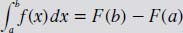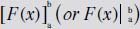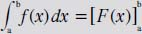NextPrevious# What is the Fundamental Theorem of the Calculus?

Chapter Mathematical Analysis

The Fundamental Theorem of the Calculus is the connection (or, more accurately, the bridge) between the integral and the derivative; in other words, it is another way of finding the area under a curve (see above) by evaluating the integral. In particular, if F(x) is a function whose derivative is f(x), then the area under the graph of y = f(x) between the points a and b is equal to F(b) - F(a). This is seen as the following notations:The number F(b) - F(a) is also denoted byThus,Close

This is a web preview of the "The Handy Math Answer Book" app. Many features only work on your mobile device. If you like what you see, we hope you will consider buying. Get the App